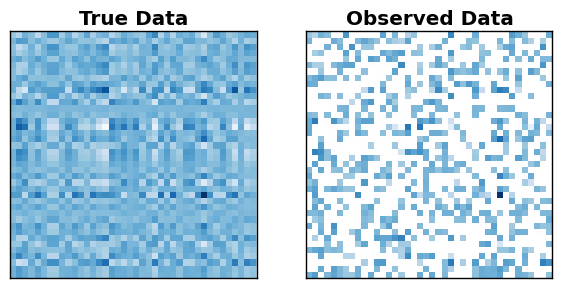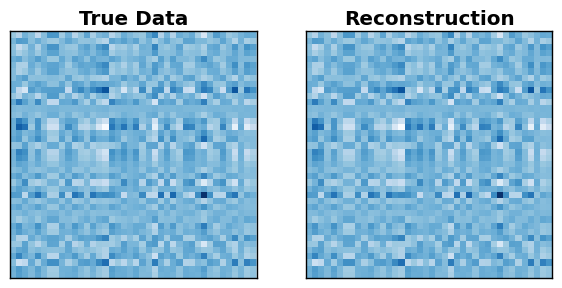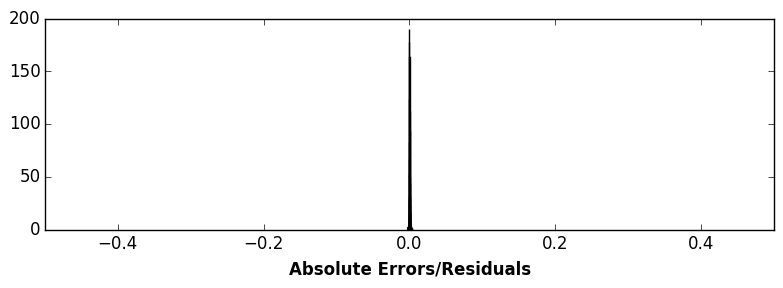# Matrix Completion in Julia via Semidefinite Programming¶

### Author: Alex Williams¶

License:### Relevent papers¶

Maryam Fazel. Matrix Rank Minimization with Applications. Stanford University, Thesis (2002).

Benjamin Recht. A Simpler Approach to Matrix Completion.. J Mach Learn. 12, 3413-3430 (2009).

Emmanuel J. Candès, Benjamin Recht. Exact Matrix Completion via Convex Optimization. Found Comput Math 9, 717–772 (2009)

Emmanuel J. Candès, Terence Tao. The Power of Convex Relaxation: Near-Optimal Matrix Completion. IEEE Transactions on Information Theory 56, 2053–2080 (2010).

Emmanuel J Candès, Yaniv Plan. Matrix Completion With Noise. Proc. IEEE 98, 925–936 (2010).

Benjamin Recht, Maryam Fazel, Pablo A. Parrilo. Guaranteed Minimum-Rank Solutions of Linear Matrix Equations via Nuclear Norm Minimization. SIAM Rev. 52, 471–501 (2010).

### Relevent Code¶

Github Repo: Convex.jl

Paper: Madeleine Udell, Karanveer Mohan, David Zeng, Jenny Hong, Steven Diamond, Stephen Boyd. Convex Optimization in Julia. SC14 Workshop on High Performance Technical Computing in Dynamic Languages

In :
using PyPlot
using Convex
using SCS

# passing in verbose=0 to hide output from SCS
solver = SCSSolver(verbose=0)
set_default_solver(solver);

INFO: Loading help data...


### Overview/Background¶

Many datasets are naturally organized into a table or matrix. Each row represents an object, event, or person under study, and each column represents a different measurement or attribute of those items. A famous example is the Netflix problem dataset. The dataset contains user ratings of Netflix movies, with each row representing a customer/user and each column representing a movie available to watch. However, each user only watches and rates very few movies, so our observations of this matrix are very incomplete. Estimating the complete matrix would help Netflix predict how individual users would rate future movies, and thus make appropriate reccomendations.

In this case, we might expect the data matrix of user ratings to be approximately low-rank, since an individual’s tastes or preferences are probably well-described by a handful of factors and interests. Other applications and motivations for low-rank matrix completion can be found in the papers above, and in this lecture by Emmanuel Candès.

### Problem Statement¶

Let $D$ denote a data matrix with many incomplete entries, and let $\Omega$ be the set of all indices in $D$ that are observed. Ideally, we would seek a reconstruction of the full matrix, $X$, as the solution to the optimization problem:

\begin{aligned} & \underset{X}{\text{minimize}} & & \text{rank}(X) & & \\ & \text{subject to} & & X_{ij} = D_{ij} & & \forall (i,j) \in \Omega \end{aligned}

However, as described in the references above, this is an NP-hard problem that is very difficult to solve directly. Instead, we solve a convex relaxation of this problem (which turns out to be a semidefinite program):

\begin{aligned} & \underset{X}{\text{minimize}} & & ||X||_* & & \\ & \text{subject to} & & X_{ij} = D_{ij} & & \forall (i,j) \in \Omega \end{aligned}

Where $||\cdot||_*$ denotes the nuclear norm of a matrix, which is simply the sum of the singular values:

$$||A||_* = \sum_{k=1}^n \sigma_k(A) = \text{trace}\left(\sqrt{A^T A}\right)$$

Penalizing the nuclear norm of our reconstructed estimate, $X$, encourages a low-rank solution. This is analogous to penalizing the $\ell_1$ norm of a reconstructed signal, which encourages the signal to be sparse. In our case, we want the singular values, $\sigma_k$, to be sparse.

### Create some synthetic data, and observe a subset of the entries¶

In :
# Construct a random m-by-n matrix matrix of rank k
m,n,k = 40,40,2
Dfull = randn(m,k)*randn(k,n) # ground truth data

# Suppose that we only observe a fraction of entries in Dfull
n_obs = 600
Dobs = fill(NaN,(m,n))
obs = randperm(m*n)[1:n_obs]
Dobs[obs] = Dfull[obs] # partially observed matrix

# Plot the ground-truth, full dataset and our partial observations
figure(figsize=(7,14))
subplot(1,2,1)
imshow(Dfull,cmap=ColorMap("Blues"),interpolation="None")
xticks([]),yticks([]),title("True Data",fontweight="bold")

subplot(1,2,2)
imshow(Dobs,cmap=ColorMap("Blues"),interpolation="None")
xticks([]),yticks([]),title("Observed Data",fontweight="bold")
show()### Solve the Semidefinite Program using Convex.jl¶

In :
# Reconstruct the full matrix, via nuclear norm minimization
Dest = Variable(m,n) # reconstructed/estimated matrix
p = minimize(nuclear_norm(Dest)) # objective function
p.constraints += norm_2(Dobs[obs] - Dest[obs]) <= 0.01 # error tolerance/constraint
solve!(p)

# Plot the ground-truth vs our reconstruction
figure(figsize=(7,14))
subplot(1,2,1)
imshow(Dfull,cmap=ColorMap("Blues"),interpolation="None")
xticks([]),yticks([]),title("True Data",fontweight="bold")

subplot(1,2,2)
imshow(Dest.value,cmap=ColorMap("Blues"),interpolation="None")
xticks([]),yticks([]),title("Reconstruction",fontweight="bold")
show()### A simple histogram of the residuals shows the matrix was exactly recovered¶

In :
figure(figsize=(8,3))
PyPlot.hist(vec(Dfull-Dest.value),100)
xlim([-0.5,0.5]),xlabel("Absolute Errors/Residuals",fontweight="bold"),tight_layout()
show()